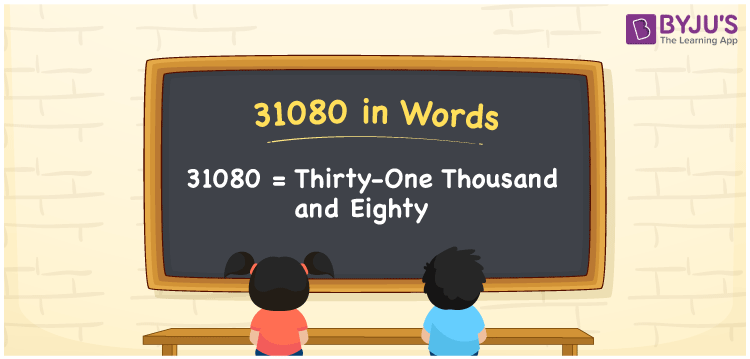31080 in words is written as Thirty-one thousand eighty. In both the International System of Numerals and the Indian System of Numerals, 31080 is written as Thirty-one thousand eighty. The number 31080 is a Cardinal Number as it could represent some quantity. For example, “the course fee is 31080 rupees”.

 31080 in Words Thirty-one thousand eighty Thirty-one thousand eighty in Number 31080

## 31080 in English Words

31080 in English words is read as “Thirty-one thousand eighty”.## How to Write 31080 in Words?

To write 31080 in words, we shall use the place value chart. In the place value chart, put 3 in the ten thousand, 1 in the thousands, 0 in the hundreds, 8 in the tens and 0 in the ones. Let us make a place value chart to write the number 31080 in words.

 Ten Thousands Thousands Hundreds Tens Ones 3 1 0 8 0

Thus, we can write the expanded form as

3 × Ten Thousand + 1 × Thousand + 0 × Hundred + 8 × Ten + 0 × One

= 3 × 10000 + 1 × 1000 + 0 × 100 + 8 × 10 + 0 × 1

= 30000 + 1000 + 0 +80 + 0

= 31080

= Thirty-one thousand eighty.

31080 is a natural number, the successor of 31079 and the predecessor of 31081.

31080 in words – Thirty-one thousand eighty

• Is 31080 an odd number? – No
• Is 31080 an even number? – Yes
• Is 31080 a perfect square number? – No
• Is 31080 a perfect cube number? – No
• Is 31080 a prime number? – No
• Is 31080 a composite number? – Yes

## Frequently Asked Questions on 31080 in Words

Q1

### How to write 31080 in words?

31080 in words is written as Thirty-one thousand eighty.
Q2

### How to write 31080 in the International and Indian System of Numerals?

In both, the system of numerals, 31080 in words, is written as Thirty-one thousand eighty.
Q3

### How to write 31080 in a place value chart?

In the place value chart, write 3 in the ten thousand, 1 in the thousands, 0 in the hundreds, 8 in the tens and 0 in the ones, respectively.